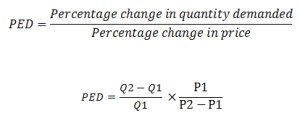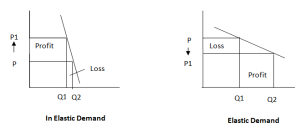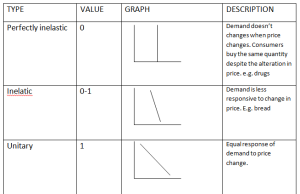Monday , June 24 2019
Home » Igcse Economics Revision Notes » Price Elasticity Of Demand

Price Elasticity Of Demand

Price Elasticity Of Demand, measures the responsiveness of demand to a change in price.

The formula used to calculate (PED) is:Q1 = Old Quantity

Q2 = New Quantity

P1 = Old Price

P2 = New Price

If the answer using the above formula is less than 1 than the product has price inelastic demand

however, if the answer is greater than 1 than the product has price elastic demand.

Price Elastic Demand: When demand changes by a greater percentage than the changes in price.

Price Inelastic Demand: When demand changes by a smaller percentage than the changes in price.

Revenue Maximization By Using Price Elasticity Of Demand:

Revenue: Total reward of producing goods and services.

Formula:

• Price/unit × Quantity produced /demanded
• Total cost + Total profitThe above diagrams show that:

If demand is inelastic, producers must charge high prices in order to maximize revenue.

If demand is elastic, producers must charge low price in order to maximize revenue.

Types Of Price Elasticity Of Demand:Factors Affecting Price Elasticity Of Demand:

Availability Of Substitutes:

Substitutes more available      PED will be elastic

Less substitutes available         PED will be in elastic

Proportion Of Income Spent:

Small proportion (e.g. salt)        PED will be inelastic

Large proportion (e.g. car)         PED will be elastic

Nature Of Product:

Need (e.g. bread)       PED will be inelastic

Luxuries (e.g. car)       PED will be elastic

Cigarettes are addictive thus it will have inelastic PED.

Fashion and Trend:

In fashion             PED will be elastic

Out of fashion     PED will be inelastic